# Nonconstant-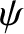Linear Resonant Response Regimes

Suppose that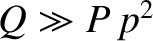and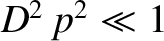. It follows that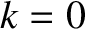,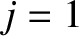,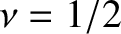, and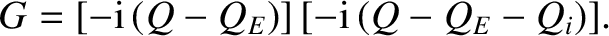(5.103)

Hence, we deduce that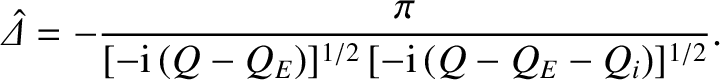(5.104)

This response regime is known as the inertial regime, because the layer response is dominated by ion inertia [2,13]. Note that the plasma response in the inertial regime is equivalent to that of two closely-spaced shear-Alfvén resonances that straddle the rational surface . In fact, it is easily demonstrated that in real space,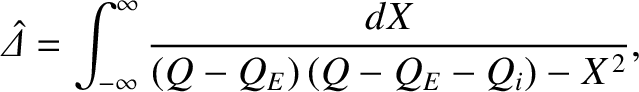(5.105)

which suggests that the resonances lie at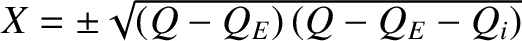. The characteristic layer width is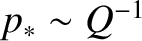, which implies that the regime is valid when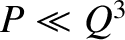,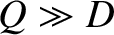,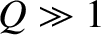, and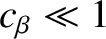.

Suppose that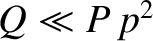and. It follows that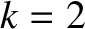,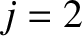,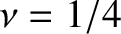, and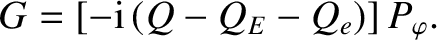(5.106)

Hence, we deduce that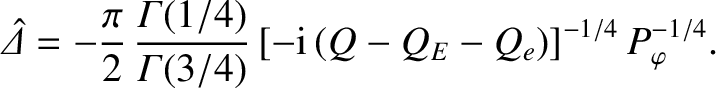(5.107)

This response regime is known as the viscous-inertial regime, because the layer response is dominated by ion perpendicular viscosity and ion inertia . The characteristic layer width is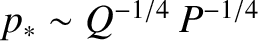, which implies that the regime is valid when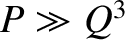,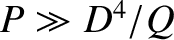,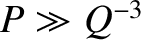, and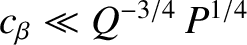.

Suppose, finally, thatand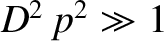. It follows that,,, and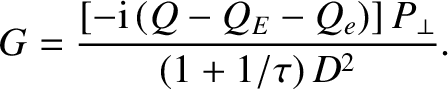(5.108)

Hence, we deduce that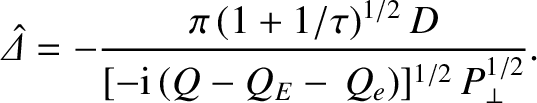(5.109)

This response regime is known as the diffusive-inertial regime, because the layer response is dominated by perpendicular energy diffusivity and ion inertia . The characteristic layer width is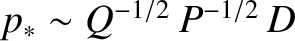, which implies that the regime is valid when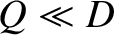,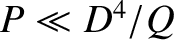,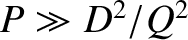, and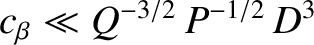.